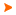Podaci o VU Početna stranicaArhiva nastavnih programaArhiva ak. kalendaraAbbreviation: B11A01 Load: 30(L) + 0(P) + 0(FE) + 0(LE) + 0(S) + 0(PEE) + 30(E) + 0(DE)
 Lecturers in charge: doc. dr. sc. Jelka Beban-Brkić Lecturers: v. pred. mr. sc. Željka Tutek ( Exercises ) Course description: Recognize the acquired mathematical and numerical skills of analytical geometry and linear algebra in the field of study. Use of acquired mathematical and numerical skills of analytical geometry and linear algebra to solve problems in the field of study.Learning outcomes at the level of the programme to which the course contributesDemonstrate competences in theoretical principles, procedures of computing and visualising the surveying data.Understand mathematical methods and physical laws applied in geodesy and geoinformatics.Apply knowledge of mathematics and physics for the purpose of recognizing, formulating and solving of problems in the field of geodesy and geoinformatics.Exercise appropriate judgements on the basis of performed calculation processing and interpretation of data obtained by means of surveying and its results.Take responsibility for continuing academic development in the field of geodesy and geoinformatics, or related disciplines, and for the development of interest in lifelong learning and further professional education.Learning outcomes expected at the level of the courseMaster the fundamental vector algebra and analytic geometry concepts and apply them in solving tasks;Identify and differentiate between types of second order surfaces;Explain the concepts of matrices and determinants, list their properties and use them in computations with matrices and determinants;Distinguish methods for solving systems of linear equations and apply the appropriate method to solve a given system;Describe the method of least squares and argue its application in solving tasks;Define the terms of eigenvalues and eigenvectors and know their typical applications;Describe and implement the concepts of diagonalization and orthogonal diagonalization of a matrix.Use the system for e-learning.Course content broken down in detail by weekly class schedule (syllabus)Vector algebra. 3hAnalytical geometry. 3hEquation, sketch and recognition of surfaces of the second order. 1hMatrix algebra. 2hElementary transformations and elementary matrices. 1hReview of previous work. 1h1st preliminary exam 1hReduced form of the matrix, inverse matrix. 2hSolving linear systems using the Gauss-Jordan reduction. Homogeneous linear systems. The Kronecker-Capelli theorem. 2hThe concept and calculation of determinants. Cramer's rule. 2hLeast squares method. 1hReview of previous work. 1h2nd preliminary exam 1hVector space. Linear independence. 2hCoordinates and change of basis. Eigenvalues and eigenvectors. 2hLinear transformations. Matrix diagonalization. 2hQuadratic forms. Diagonalization of quadratic forms. 2hThe final exam. 1hScreening student workClass attendance - Requirement for the signatureindependent assignments - 4%interactive tasks - 4%Tests - 92%Oral exam - optionalWritten exam - 100%
 Lecture languages: - - -
 Compulsory literature: 1. Beban Brkić, J., Tutek, Ž.: Analitička geometrija i linearna algebra, Interna skripta, dostupna na e-učenju Elezović, N.: Linearna algebra, Element, Zagreb 2003. Elezović, N., Aglić, A.: Linearna algebra, Zbirka zadataka, Element, Zagreb 2003.
 Recommended literature: 2. Anton, H., Rorres, C.: Elementary Linear Algebra, John Wiley & Sons, Inc., N. Y. 2000. Internetske aplikacije za vježbu.Legend L - Lectures P - Practicum FE - Field exercises LE - Laboratory exercises S - Seminar PEE - Physical education excercises. E - Exercises DE - Design exercises * - Not graded| Početna stranica | Visoka učilišta | Korisničke stranice | | Na vrh | Copyright (c) 2006. Ministarstva znanosti, obrazovanja i športa. Sva prava zadržana. Programska podrška (c) 2006. Fakultet elektrotehnike i računarstva. Oblikovanje(c) 2006. Listopad Web Studio. Posljednja izmjena 2017-09-26